# Year 9 Revision - Level 1

This is a level 1 revision exercise to help prepare for Year 9/end of Key Stage 3 tests.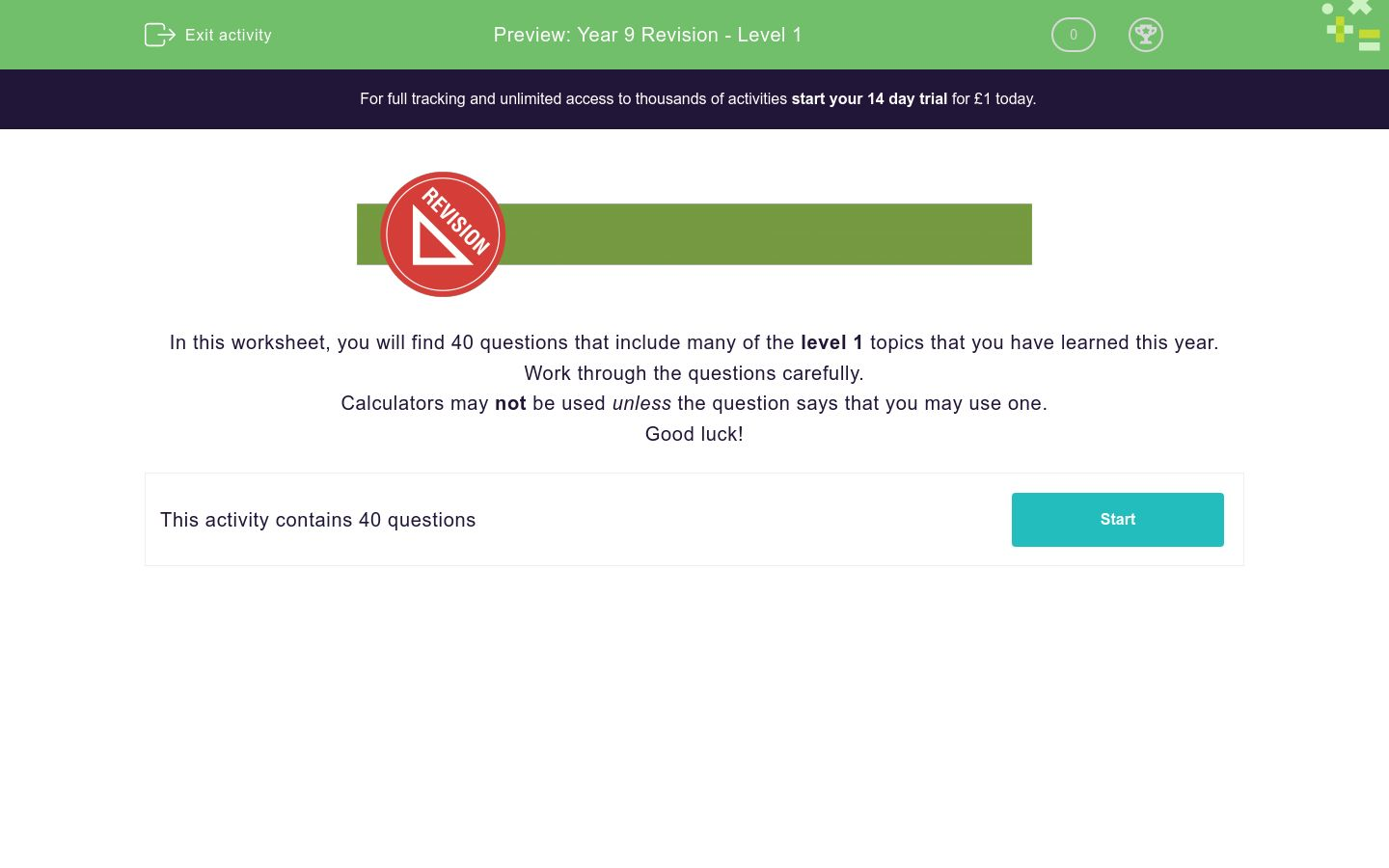Key stage:  KS 3

Curriculum topic:   Revision

Curriculum subtopic:   Year 9 Revision

Difficulty level:### QUESTION 1 of 10In this worksheet, you will find 40 questions that include many of the level 1 topics that you have learned this year.

Work through the questions carefully.

Calculators may not be used unless the question says that you may use one.

Good luck!

Work out:

840.3 + 8.403 + 84.03

Work out:

14.3 × 8.4

In a room of people:

½ of them wear glasses.

¼ of them wear contact lenses.

The rest have perfect eyesight.

What percentage have perfect eyesight?

(Just write the number.)

Is the following number divisible by 11?

42 141

yes

no

Multiples of 10.

Is this list finite or infinite?

finite

infinite

Round the following number to 1, 2 and 3 significant figures.

4225

## Column B

1 sig. fig.
4200
2 sig. figs.
4230
3 sig. figs.
4000

Round the following number to 2 decimal places.

42.259

Write the following number in standard form:

8051

80.51 × 102

8.051 × 102

8.051 × 103

Work out:

 15 + 5 40 20

(Give your answer as a fraction, reduced to its lowest terms, e.g. 3/10.)

Work out:

 7 ÷ 5 40 20

(Give your answer as a fraction, reduced to its lowest terms, e.g. 3/10.)

Match the fractions to their decimal equivalents.

## Column B

1/10
0.25
1/2
0.1
1/4
0.5
3/4
0.75

Work out:

0.024 ÷ 0.04

Work out:

 ( 1 )3 4

Round each of these numbers to 1 significant figure to work out an approximate answer to:

389 × 19.5

Work out:

12% of 35

Find the original price of a television in pounds, sold for £350 after a 50% reduction in a sale.

(Just write the number.)You may use a calculator for this question.

Jane sells 110 cakes on Monday.

She sells 132 cakes on Tuesday.

What is the percentage increase in sales from Monday to Tuesday?

(Just write the number.)

Work out the nth term in this sequence:

4, 7, 10, 13, 16 ...

n + 3

3n + 1

4n + 3

4n + 7

What is the sum of the angles shown in this triangle?

(Just write the expression. No need to include the degrees sign.)Simplify:

(5a + 1) - (5a - 1)

Simplify:

2a3 × 3a2

6a5

5a6

6a6

Multiply out the brackets and simplify:

3x - 8(1 - 4x)

-8 - 29x

35x - 8

x - 8

Simplify:

(b3)2

b9

b32

b6

Solve for a:

6a - 2 = 2a - 22

a = -6

a = 24

a = -5

Solve the inequality:

6x + 4 ≤ -20

x < 3

x ≥ 4

x ≤ -4

x < -4

Work out the value of the following expression when a = -16 and b = 72.

 a + b 2 8

Find the gradient of the red line.

(If it is not a whole number, write it as a fraction in the form a/b.)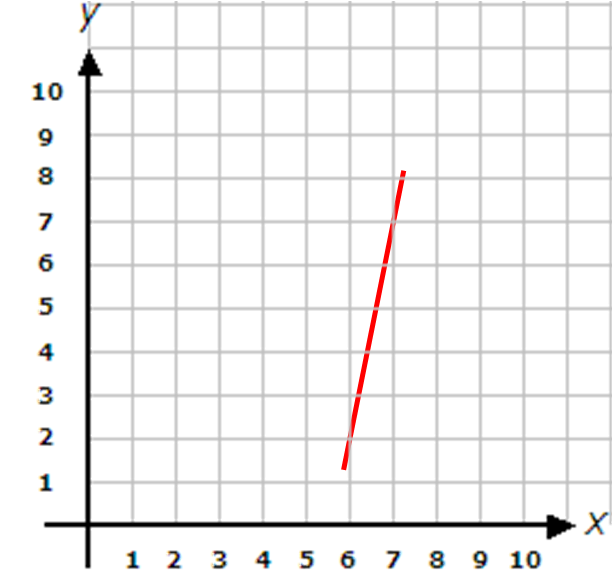Look at this grid.

What are the coordinates of G after it has been reflected in the y-axis?

(Write your answer in the form (a, b) using brackets and a comma between the coordinate numbers.)A sum of money is shared in the ratio 5:3 between two people, A and B.

If A gets £95, how much does B get in £?

(Just write the number.)

John sets off on a car journey at 08:15.

He travels at an average speed of 85 km/h and arrives at his destination at 10:45.

Find the distance he travelled in km.

(Just write the number.)You may use a calculator for this question.

Find the area in cm2 of a circle of radius 6 cm.

(Just write the number to 1 decimal place.)You may use a calculator for this question.

Find the volume of this cylinder in cm3 to the nearest whole number.

Remember that the volume = base area × height.

(Just write the whole number.)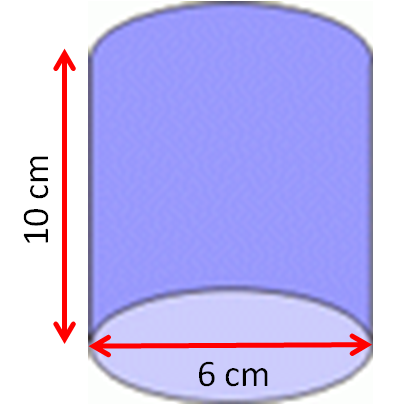A regular polygon has 8 sides.

Calculate its exterior angle.

(Just write the number.)You may use a calculator for this question.

Use Pythagoras' Theorem to calculate the length of the hypotenuse, c, in the following right-angled triangle.In relation to the angle θ, label the sides correctly in this right-angled triangle.## Column B

a
opposite
c
hypotenuse
b

Which trigonometric ratio is used to calculate the angle θº ?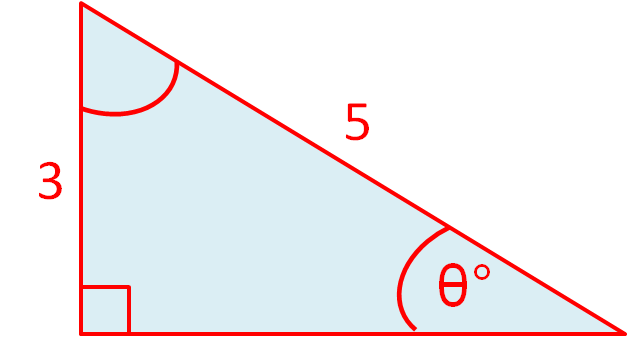sin

cos

tan

One fair coin and one fair die are thrown.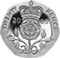Make a list of the possible outcomes on a piece of paper.

In how many outcomes is there a tail and an even number?

The following table shows the numbers of right- and left-handed children in a class of 30 children.

Boys Girls Total
Right 10 12 22
Left 5 3 8
Total 15 15 30

What is the probability that a child chosen at random is a right-handed girl?

(Write your answer with the / symbol, e.g. 2/3 and remember to reduce your fraction to its lowest terms.)

Work out the mean, mode, median and range of the following set of numbers:

3,  15,  3,  12,  4.5

Which line is the line of best fit on this scatter graph?A

B

C

• Question 1

Work out:

840.3 + 8.403 + 84.03

932.733
EDDIE SAYS
Line up the decimal points, and add the digits in columns.
• Question 2

Work out:

14.3 × 8.4

120.12
EDDIE SAYS
Use long multiplication to work out 143 × 84.
Then divide the answer by 100.
• Question 3

In a room of people:

½ of them wear glasses.

¼ of them wear contact lenses.

The rest have perfect eyesight.

What percentage have perfect eyesight?

(Just write the number.)

25
EDDIE SAYS
¼ of the people in the room have perfect eyesight.
¼ of 100 = 25.
• Question 4

Is the following number divisible by 11?

42 141

yes
EDDIE SAYS
4 + 1 + 1 = 6
2 + 4 = 6, which matches.
• Question 5

Multiples of 10.

Is this list finite or infinite?

infinite
EDDIE SAYS
Finite lists have a set number of items.
Infinite lists go on for ever.
Lists of multiples go on for ever, because you can always find the next multiple.
• Question 6

Round the following number to 1, 2 and 3 significant figures.

4225

## Column B

1 sig. fig.
4000
2 sig. figs.
4200
3 sig. figs.
4230
EDDIE SAYS
1 sig. fig: 4225 is between 4000 and 5000 but nearer to 4000.
2 sig. figs: 4225 is between 4200 and 4300 but nearer to 4200.
3 sig. figs: 4225 is between 4220 and 4230 but 'nearer' to 4230, as halfway numbers always round up.
• Question 7

Round the following number to 2 decimal places.

42.259

42.26
EDDIE SAYS
42.259 is between 42.25 and 42.26, but it is nearer to 42.26.
• Question 8

Write the following number in standard form:

8051

8.051 × 103
EDDIE SAYS
Fix the decimal point after the first digit, then count how many times you must multiply by 10 to get up to 8051.
• Question 9

Work out:

 15 + 5 40 20

(Give your answer as a fraction, reduced to its lowest terms, e.g. 3/10.)

5/8
EDDIE SAYS
15/40 + 10/40 = 25/40 = 5/8
• Question 10

Work out:

 7 ÷ 5 40 20

(Give your answer as a fraction, reduced to its lowest terms, e.g. 3/10.)

7/10
EDDIE SAYS
Turn second fraction upside down and multiply.
7/40 × 20/5
Reduce to get 7/10.
• Question 11

Match the fractions to their decimal equivalents.

## Column B

1/10
0.1
1/2
0.5
1/4
0.25
3/4
0.75
EDDIE SAYS
1/10 = 0.1
1/2 = 5/10 = 0.5
1/4 = 25/100 = 0.25
3/4 = 75/100 = 0.75
• Question 12

Work out:

0.024 ÷ 0.04

0.6
EDDIE SAYS
Multiply both numbers by 100 to get:
2.4 ÷ 4 = 0.6
• Question 13

Work out:

 ( 1 )3 4

1/64
EDDIE SAYS
1/4 × 1/4 × 1/4
• Question 14

Round each of these numbers to 1 significant figure to work out an approximate answer to:

389 × 19.5

8000
EDDIE SAYS
400 × 20 = 8000
• Question 15

Work out:

12% of 35

4.2
EDDIE SAYS
0.12 × 35 = 4.2
• Question 16

Find the original price of a television in pounds, sold for £350 after a 50% reduction in a sale.

(Just write the number.)

700
EDDIE SAYS
In a 50% sale, prices are halved.
350 ÷ 0.5 = 350 × 2 = 700
• Question 17You may use a calculator for this question.

Jane sells 110 cakes on Monday.

She sells 132 cakes on Tuesday.

What is the percentage increase in sales from Monday to Tuesday?

(Just write the number.)

20
EDDIE SAYS
Actual increase = 132 - 110 = 22
% increase = 22 ÷ 110 × 100 = 20
• Question 18

Work out the nth term in this sequence:

4, 7, 10, 13, 16 ...

3n + 1
EDDIE SAYS
Common difference is 3.
• Question 19

What is the sum of the angles shown in this triangle?

(Just write the expression. No need to include the degrees sign.)10a+70
10a + 70
70+10a
70 + 10a
EDDIE SAYS
5a + 3a + 30 + 2a + 40 = 5a + 3a + 2a + 30 + 40 = 10a + 70
• Question 20

Simplify:

(5a + 1) - (5a - 1)

2
EDDIE SAYS
5a + 1 - 5a + 1
• Question 21

Simplify:

2a3 × 3a2

6a5
EDDIE SAYS
2 × 3 × a × a × a × a × a
• Question 22

Multiply out the brackets and simplify:

3x - 8(1 - 4x)

35x - 8
EDDIE SAYS
3x - 8 + 32x
• Question 23

Simplify:

(b3)2

b6
EDDIE SAYS
b3 × b3
• Question 24

Solve for a:

6a - 2 = 2a - 22

a = -5
EDDIE SAYS
6a - 2a = 2 - 22
4a = -20
a = -5
• Question 25

Solve the inequality:

6x + 4 ≤ -20

x ≤ -4
EDDIE SAYS
6x ≤ -24
• Question 26

Work out the value of the following expression when a = -16 and b = 72.

 a + b 2 8
1
EDDIE SAYS
-8 + 9
• Question 27

Find the gradient of the red line.

(If it is not a whole number, write it as a fraction in the form a/b.)5
EDDIE SAYS
5 up for every 1 to the right.
• Question 28

Look at this grid.

What are the coordinates of G after it has been reflected in the y-axis?

(Write your answer in the form (a, b) using brackets and a comma between the coordinate numbers.)(-2,3)
(-2, 3)
EDDIE SAYS
The x-coordinate changes its sign from 2 to -2.
The y-coordinate stays at 3.
• Question 29

A sum of money is shared in the ratio 5:3 between two people, A and B.

If A gets £95, how much does B get in £?

(Just write the number.)

57
EDDIE SAYS
There are £95 in 5 bags, so each bag contains £95 ÷ 5 = £19.
B gets 3 bags, i.e. 3 × £19 = £57.
• Question 30

John sets off on a car journey at 08:15.

He travels at an average speed of 85 km/h and arrives at his destination at 10:45.

Find the distance he travelled in km.

(Just write the number.)

212.5
EDDIE SAYS
His journey is 2½ hours long.
85 × 2 = 170
½ of 85 = 42.5
170 + 42.5 = 212.5
• Question 31You may use a calculator for this question.

Find the area in cm2 of a circle of radius 6 cm.

(Just write the number to 1 decimal place.)

113.1
EDDIE SAYS
Area = π × r2 = π × 36 = 113.097...
• Question 32You may use a calculator for this question.

Find the volume of this cylinder in cm3 to the nearest whole number.

Remember that the volume = base area × height.

(Just write the whole number.)283
EDDIE SAYS
π × 3² × 10 = 282.74...
• Question 33

A regular polygon has 8 sides.

Calculate its exterior angle.

(Just write the number.)

45
EDDIE SAYS
360 ÷ 8
• Question 34You may use a calculator for this question.

Use Pythagoras' Theorem to calculate the length of the hypotenuse, c, in the following right-angled triangle.10.4
EDDIE SAYS
Work out 32 + 102 = 109.
√109 = 10.440...
• Question 35

In relation to the angle θ, label the sides correctly in this right-angled triangle.## Column B

b
opposite
a
hypotenuse
c
EDDIE SAYS
a is opposite the angle θ.
b is next to or adjacent to the angle θ.
c is the longest side.
• Question 36

Which trigonometric ratio is used to calculate the angle θº ?sin
EDDIE SAYS
sin θ = opposite / hypotenuse
• Question 37

One fair coin and one fair die are thrown.Make a list of the possible outcomes on a piece of paper.

In how many outcomes is there a tail and an even number?

3
EDDIE SAYS
T2, T4, T6
• Question 38

The following table shows the numbers of right- and left-handed children in a class of 30 children.

Boys Girls Total
Right 10 12 22
Left 5 3 8
Total 15 15 30

What is the probability that a child chosen at random is a right-handed girl?

(Write your answer with the / symbol, e.g. 2/3 and remember to reduce your fraction to its lowest terms.)

2/5
EDDIE SAYS
12/30 reduces to 2/5.
• Question 39

Work out the mean, mode, median and range of the following set of numbers:

3,  15,  3,  12,  4.5

EDDIE SAYS
Mean = (3 + 15 + 3 + 12 + 4.5) ÷ 5 = 37.5 ÷ 5 = 7.5
Mode is most frequently occuring.
Median is middle of ordered list: 3, 3, 4.5, 7.5, 12.
Range = largest - smallest = 15 - 3 = 12
• Question 40

Which line is the line of best fit on this scatter graph?C
EDDIE SAYS
The line C goes through the most points and follows the general direction of the points.
---- OR ----

Sign up for a £1 trial so you can track and measure your child's progress on this activity.

### What is EdPlace?

We're your National Curriculum aligned online education content provider helping each child succeed in English, maths and science from year 1 to GCSE. With an EdPlace account you’ll be able to track and measure progress, helping each child achieve their best. We build confidence and attainment by personalising each child’s learning at a level that suits them.

Get started